MzChronogram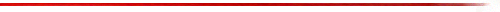### SYNOPSIS

MzChronogram -- Time-domain analogy to spectrograms.
The MzChronogram plugin displays a time-domain signal in two dimensions as if it were a set of frequency frames in a spectrogram. The time sequence runs from top to bottom, from left to right. Stable periodic sounds generate displays of parallel lines with the line slope dependent on the frequencies of the sinewaves.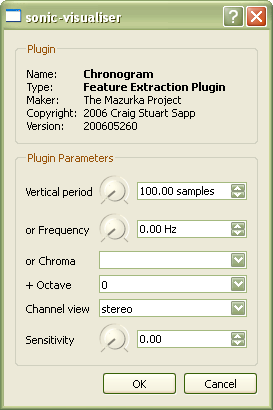### INPUT PARAMETERS

MzChronogram accepts 6 input parameters:

1. Vertical period
The pixel height of each channel in terms of the number of audio samples to display in each data column.
2. Frequency
The height of each channel display in terms of a frequency (inverse of period). If this value is changed from its default setting, then the plugin will use this value instead of the vertical period parameter.
3. Chroma
The pitch-class for a frequency height specification. If the chroma is set to a value other than "none", then the vertical period will be calculated from the fundamental frequency of the specified pitch-class at the given octave (overriding both the vertical period and frequency parameters).
4. Octave
Used in conjunction with the chroma parameter. Octave 4 is the octave of middle C (C4). Frequencies are calculated from the chroma/octave values using equal-temperament with A4=440 Hz.
5. Channel view
By default, all audio channels will be displayed in the chronogram. You can also use this parameter to display only the left channel, right channel or the stereo difference.
6. Sensitivity
A parameter which controls the contrast between low amplitude and high amplitude parts of the audio data.

### DESCRIPTION

The following diagram demonstrates how the sound samples are filled into a two-dimensional grid in the chronographic display. The audio is split up into frames equal to the number of rows in the chronogram. Then the frame column is filled with audio sample data from the top to the bottom of the column. In Sonic Visualiser, the chronogram columns line-up exactly with the corresponding display of the audio waveform.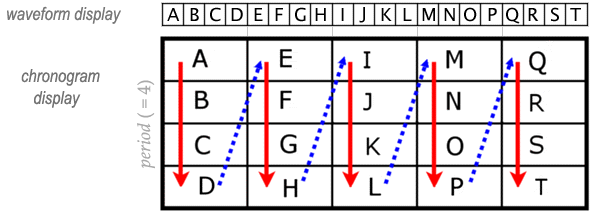When a sound contains a period which is an exact multiple of the vertical period of the chronographic display, that sound will be displayed as horizontal lines on the display as shown in the figure below. In this example, there is a sinewave oscillator which plays 43 Hz for one second, then sweeps up to 5 * 43 = 215 Hz and stays there for one second, then sweeps up to 10 * 43 = 430 Hz and stay there for one second. In each case where the frequency is a multiple of the sampling rate divided by the vertical period of the chronographic display, horizontal lines will be displayed. The number of horizontal lines indicates the frequency of the pitch. For example, the middle of the picture where there are 5 red bands indicates a frequency of 5 * 44100/1024 = 215 Hz.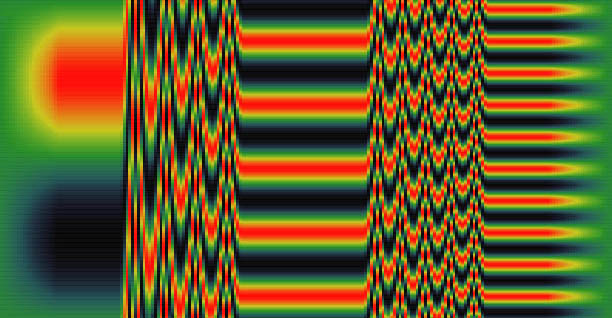Stable frequencies which are not multiples of the vertical period of the chronogram will form parallel lines at various angles. Lines which slope upwards are slightly sharper than the horizontal frequency represented by the number of vertical lines. And likewise, falling slopes indicate a flatter frequency. The following example starts at 10 times the frame frequency of 43 Hz, and then increases the pitch by 43/8 Hz until the oscillator arrives at 11 times the frame frequency (count the number of red lines at the right side of the picture).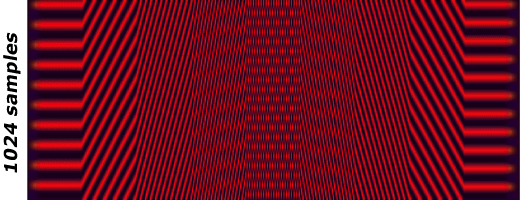For complex harmonic tones which contain more than one sinewave, the pitch of the tone at its fundamental frequency is visible as a slope of parallel lines. Each of the harmonics of the tone are on a similar slope. For example the first overtone occurs twice as often as the fundamental frequency, so their periods match at 2:1. Likewise, the second overtone at an octave and a fifth above the fundamental frequency has a ratio of 3:1 with the fundamental.

Notes which are considered consonant have similar slopes in the lines for their pitches, while notes of dissonant intervals will have dissimilar slopes. Notice in the following example that the notes "f", "a", and "c" which form a major triad all have the same primary slope.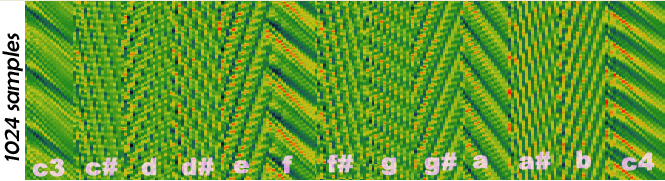Below is an example from a real performance. The original recording is in stereo, and the chronographic display will display each channel of the signal on top of each other, with the left channel above the right, as is done with the waveform display in Sonic Visualiser. In this example, you can see loud notes as regions of high contrast in the chronographic display since the waveform is oscillation between the extreme ranges of the display. Also, the chronographic display is useful to view changes of pitch where the amplitude of the waveform does not change significantly. For example, there is a low note played softly near the middle of the following diagram. The low note is visible in the chronogram as a region of parallel lines which are fairly widely spaced.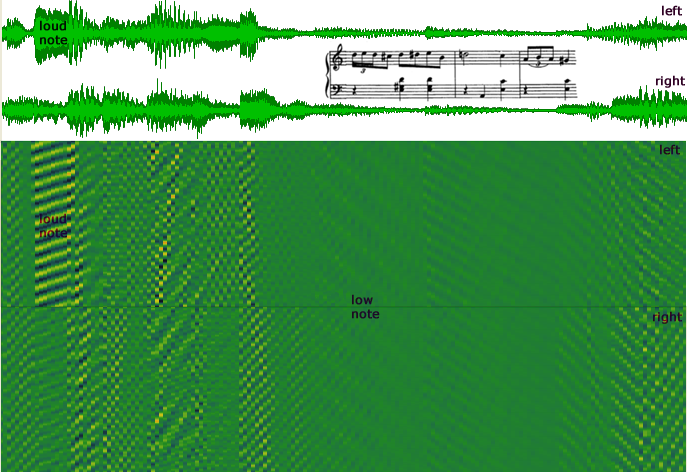```
```

Sensitivity parameter

The sensitivity parameter control for MzChronogram is used to enhance visibility in quiet regions of audio. Normally when display a region which has low amplitude, the color of the display will have a low contrast since the colorization of the display also takes into account loud sections of the audio. However, the sensitivity parameter enhances the quiet regions of the audio so that pitch banding is more visible as demonstrated in the following figure.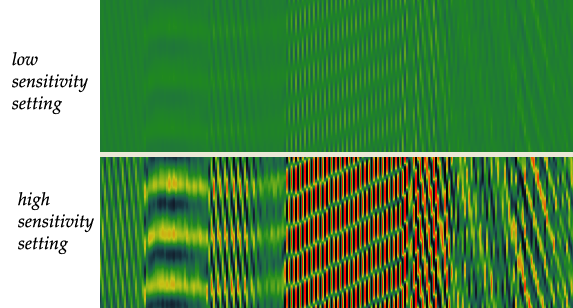Here is a plot of the sensitivity mapping which is used. When the sensitivity is set to 0.00, the waveform amplitudes are unchanged. As the sensitivity factor is raised to 1.0, low amplitude values are increased, while high amplitude values stay (mostly) the same.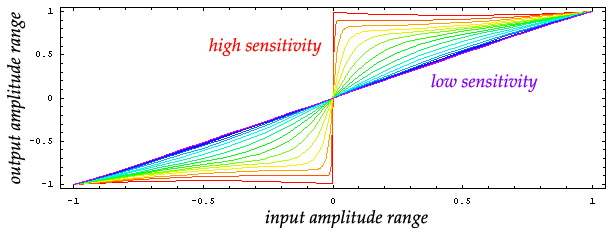### LIMITATIONS

Currently only allows for integer periods, so not all frequencies can be represented as horizontal lines. Will add a non-integer correction factor sometime in the future...

```